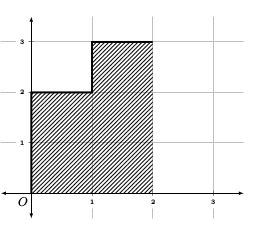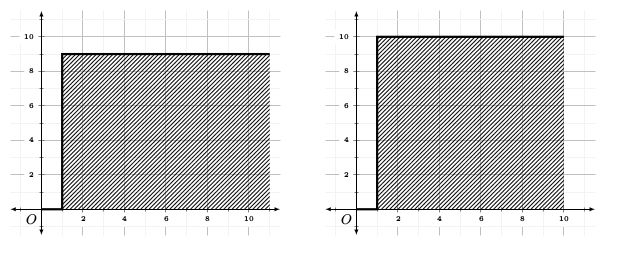시간 제한 메모리 제한 제출 정답 맞은 사람 정답 비율
1 초 512 MB 0 0 0 0.000%

## 문제

Basia has a string $s$, each character being one of the first 16 lowercase letters of the English alphabet.

Each character of this string will be replaced by an arrow to the right or up, but the same letters have to be replaced by the same arrow. For example, the string "banan" can be replaced to $\uparrow \uparrow \rightarrow \uparrow \rightarrow$ or $\uparrow \uparrow \uparrow \uparrow \uparrow$, but you cannot obtain $\rightarrow \rightarrow \rightarrow \uparrow \rightarrow$ because it would require replacing two letters 'a' with different arrows.

Basia will use the resulting sequence of arrows to draw a broken line. She will start with a pencil set at point $(0, 0)$, then $n$ times she will move it $1$ unit right or up -- in the direction of the next arrow.

As a result of this drawing we will denote the area between the broken line and the OX axis. Formally, this area is a set of points $(x, y)$ such that $y \geq 0$ and there is a point $(x, y')$ that belongs to the broken line and $y' \geq y$ occurs.

What is the largest possible result of Basia's drawing?

## 입력

The first and the only line of the standard input contains one string $s$ ($1 \leq |s| \leq 300\,000$), consisting of lowercase letters of the English alphabet 'a'-'p' (16 possible characters).

## 출력

Output one integer -- the largest possible result of the drawing obtained after conversion from letters to arrows using given rules.

## 예제 입력 1

banan


## 예제 출력 1

5


## 예제 입력 2

abcdefghijklmnopaaaa


## 예제 출력 2

90


## 힌트

String "banan" should be replaced with $\uparrow \uparrow \rightarrow \uparrow \rightarrow$. The area under the broken line is then $5$:For string "abcdefghijklmnopaaaa" there are two optimal solutions with the area 90: